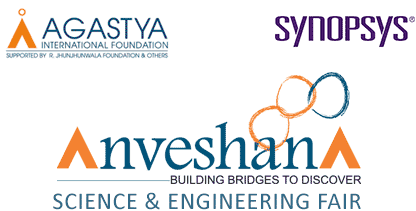### PRIZES - BANGALORE

The Prize money that will be awarded to the competitors are as follows.

 First Prize Rs. 30,000/- Second Prize Rs. 25,000/- Third Prize Rs. 20,000/- Fourth Prize Rs. 15,000/- Fifth Prize Rs. 10,000/-

5 Consolation Prize of Rs. 5000/- each.

### Special Prize

Best Hand Made Model – Rs. 5,000/-
Viewer's Choice – Rs. 5000/-

## PRIZES - North Karnataka

The Prize money that will be awarded to the competitors are as follows.

 First Prize Rs. 15,000/- Second Prize Rs. 12,000/- Third Prize Rs. 10,000/- Fourth Prize Rs. 7,500/- Fifth Prize Rs. 5,000/-

5 Consolation Prize of Rs. 2000/- each.

## PRIZES – NCR-DELHI

The Prize money that will be awarded to the competitors are as follows.

 First Prize Rs. 30,000/- Second Prize Rs. 25,000/- Third Prize Rs. 20,000/- Fourth Prize Rs. 15,000/- Fifth Prize Rs. 10,000/-

5 Consolation Prize of Rs. 5000/- each.

The Prize money that will be awarded to the competitors are as follows.

 First Prize Rs. 30,000/- Second Prize Rs. 25,000/- Third Prize Rs. 20,000/- Fourth Prize Rs. 15,000/- Fifth Prize Rs. 10,000/-

5 Consolation Prize of Rs. 5000/- each.

## PRIZES - Chittoor

The Prize money that will be awarded to the competitors are as follows.

 First Prize Rs. 15,000/- Second Prize Rs. 12,000/- Third Prize Rs. 10,000/- Fourth Prize Rs. 7,500/- Fifth Prize Rs. 5,000/-

5 Consolation Prize of Rs. 2000/- each.

## PRIZES – MUMBAI

The Prize money that will be awarded to the competitors are as follows.

 First Prize Rs. 30,000/- Second Prize Rs. 25,000/- Third Prize Rs. 20,000/- Fourth Prize Rs. 15,000/- Fifth Prize Rs. 10,000/-

5 Consolation Prize of Rs. 5000/- each.